MODELLING BISTABILITY IN TELECOMMUNICATIONS NETWORKS

by

Phil Pollett

Department of Mathematics
The University of Queensland

A SYMMETRIC FULLY-CONNECTED NETWORK

N - nodes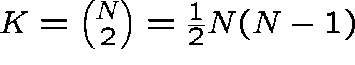- links

C - circuits on each link

Poisson traffic offered at rate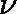Exponentially distributed holding times (mean 1)

THE GHK MODEL

Letbe the number of links with j circuits in use at time t (for a network with K links).

Let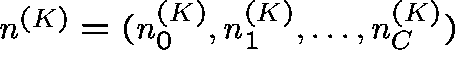. Then,is a continuous-time Markov chain which takes values in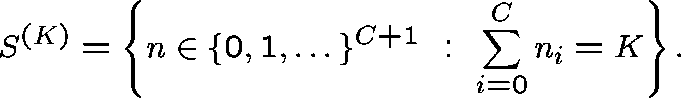The transition rates,,, of the process are given by,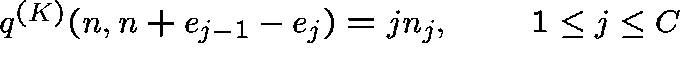,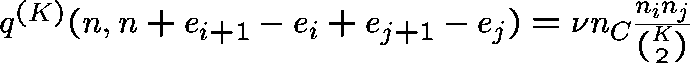,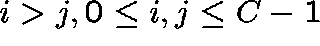,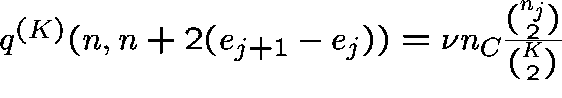,,

whereis the unit vector with 1 as its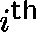entry.

THE BEHAVIOUR AS THE NETWORK BECOMES LARGE

Let, whereis the proportion of links with j circuits in use at time t.

If, as,, then, where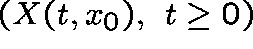is a deterministic process with initial point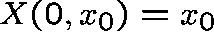and which satisfies,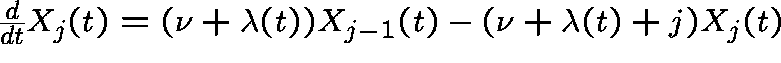,,

where.

THE EQUILIBRIUM POINTS

Ifis an equilibrium point it must be of the form given bywhere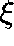solves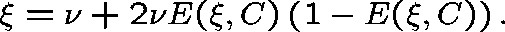The quantity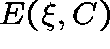, given by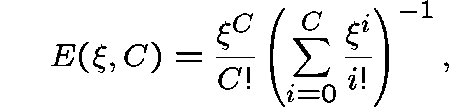is Erlang's formula for the loss probability of a single link with C circuits and with Poisson traffic offered at rate. It is usually more convenient to calculate the equilibrium points by setting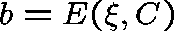and solving the equation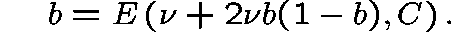MODELLING RANDOM FLUCTUATIONS

The central limit law shows that the random fluctuations about any given equilibrium point,, are Gaussian. Moreover, it shows that these fluctuations can be approximated by an Ornstein-Uhlenbeck (OU) process.

Letbe an equilibrium point. Then, ifthe family of processes, defined byconverges weakly to an Ornstein-Uhlenbeck process with initial valueand with a local drift matrix, B, and a local covariance matrix, G, which can be determined from the parameters of the model.

In particular,is normally distributed with meanand covariance matrixwhere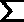, the stationary covariance matrix, satisfies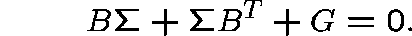We can conclude that, for K large,has an approximate normal distribution for each s, and an approximation for the mean and the covariance matrix ofis given byand